•#### Learning Bayesian Models with R#### Overview of this book

Learning Bayesian Models with RCreditswww.PacktPub.comPrefaceFree Chapter
Introducing the Probability TheoryThe R EnvironmentIntroducing Bayesian InferenceMachine Learning Using Bayesian InferenceBayesian Regression ModelsBayesian Classification ModelsBayesian Models for Unsupervised LearningBayesian Neural NetworksBayesian Modeling at Big Data ScaleIndex## Two-layer neural networks

Let us look at the formal definition of a two-layer neural network. We follow the notations and description used by David MacKay (reference 1, 2, and 3 in the References section of this chapter). The input to the NN is given by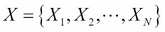. The input values are first multiplied by a set of weights to produce a weighted linear combination and then transformed using a nonlinear function to produce values of the state of neurons in the hidden layer: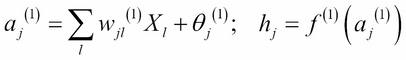A similar operation is done at the second layer to produce final output values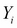: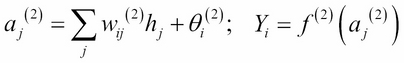The function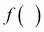is usually taken as either a sigmoid function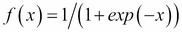or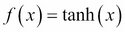. Another common function used for multiclass classification is softmax defined as follows: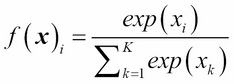This is a normalized exponential function.

All these are highly nonlinear functions exhibiting the property that the output value has a sharp increase as a function of the input. This nonlinear property gives neural networks more computational flexibility than standard linear or generalized linear models...